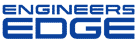# Re: Deflection of aluminum plate 3/4 inch thick

[ Home ][ Forum Archive ] [ Active Engineering Forum ]

Posted By<" ">Colin Pitts on December 20, 2001 at 15:32:16:

In Reply to: deflection of aluminum plate posted byNigel Cotton on December 19, 2001 at 16:57:41:

: Can anyone help me with this. I have a 3/4" thick aluminum plate, 20" by 16". It has atmospheric pressure on one side and vacuum on the other, the pressure on the plate being 15 pounds per square inch. How do I calculate the maxumim deflection at the center of the plate?

: I would love to have a formula to understand how to do it,

Hi Nigel,

The deflection of your plate will depend on how it's edges are supported. It is thick enough that it will not behave as a membrane and wide enough that it will be stiffer than a beam with a distributed load. (due to poisson's ratio)

The formula to use in this case is

Y=K*(W*R^4)/(E*t^3).

Where
E= elastic modulus
R= long dimension
W= pressure
t= thickness

K depends on the end supports and the aspect ratio of the plate. In this case it looks like K is .0843 (to be on the safe side). This is assuming an aspect ratio of 1.5 and a poissons ratio of .3.

If you need more accuracy go to ASME handbook "Metals Engineering: Design" or Rourks and Young.

Or you could cheat and use FEA.

Later
-colin